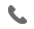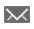•+44 7459 302492info@uplatz.com
• Register
Job Meter = High

### SSAS

30 Hours
Online Instructor-led Training
USD 1399 (USD 2800)
Save 50% Offer ends on 31-Dec-2023253 Learners

SSAS

Overview SSAS

Developing SQL Data Models course imparts core skills to implement multidimensional databases using SSAS (SQL Server Analysis Services), and create tabular semantic data models for analysis. This SSAS training is meant for database professionals who create enterprise BI solutions in order to fulfil the role of a BI Developer. This SQL Data Model Development course is also suitable for power users, information workers and data analysts.

Course Objectives

• Understand the architecture, components and features of BI solution

• Use analytics of SSAS to create a multidimensional database

• Deploy dimensions into a cube

• Deploy measures and measure groups into a cube

• Implement MDX syntax

• Perform customizations on a cube

• Create a tabular database

• Query tables using DAX

• Conduct predictive analysis using data mining

Module 1: Creating Multidimensional Databases

This module describes the steps required to create a multidimensional database with analysis

services.

Lessons

• Introduction to multidimensional analysis

• Creating data sources and data source views

• Creating a cube

• Overview of cube security

Lab: Creating a multidimensional database

After completing this module, you will be able to:

• Use multidimensional analysis

• Create data sources and data source views

• Create a cube

• Describe cube security

Module 2: Working with Cubes and Dimensions

This module describes how to implement dimensions in a cube.

Lessons

• Configuring dimensions

• Define attribute hierarchies

• Sorting and grouping attributes

Lab: Working with Cubes and Dimensions

After completing this module, you will be able to:

• Configure dimensions

• Define attribute hierarchies.

• Sort and group attributes

Module 3: Working with Measures and Measure Groups

This module describes how to implement measures and measure groups in a cube.

Lessons

• Working with measures

• Working with measure groups

Lab: Configuring Measures and Measure Groups

After completing this module, you will be able to:

• Work with measures

• Work with measure groups

Module 4: Introduction to MDX

This module describes the MDX syntax and how to use MDX.

Lessons

• MDX fundamentals

• Adding calculations to a cube

• Using MDX to query a cube

Lab: Using MDX

After completing this module, you will be able to:

• Describe the fundamentals of MDX

• Add calculations to a cube

• Query a cube using MDX

Module 5: Implementing a Tabular Data Model by Using Analysis Services

This module describes how to implement a tabular data model in PowerPivot.

Lessons

• Introduction to tabular data models

• Creating a tabular data model

• Using an analysis services tabular model in an enterprise BI solution

Lab: Working with an Analysis services tabular data model

After completing this module, you will be able to:

• Describe tabular data models

• Create a tabular data model

• Be able to use an analysis services tabular data model in an enterprise BI solution

Module 6: Introduction to Data Analysis Expression (DAX)

This module describes how to use DAX to create measures and calculated columns in a tabular data

model.

Lessons

• DAX fundamentals

• Using DAX to create calculated columns and measures in a tabular data model

Lab: Creating Calculated Columns and Measures by using DAX

After completing this module, you will be able to:

• Describe the fundamentals of DAX

• Use DAX to create calculated columns and measures in a tabular data model

--------------------------------------------------------------------------------------

# SSAS

Course Details & Curriculum
--------------------------------------------------------------------------------------